# How to Measure the Area of your Backsplash and Calculate Square Footage?

Do you want to tile your kitchen backsplash but don't know how to measure the area for the backsplash? Or maybe you're not sure how to calculate the square footage required. Don't worry. We've got you covered. This guide will walk you through the process of measuring your area with a backsplash calculator for backsplash tile and also for any space. It can be tricky to get the measurements right, but you'll have no trouble at all with this guide. We'll also provide a handy backsplash calculator to make things easier for you. So, if you are looking for pro tips, read on!## How to Measure Area For Backsplash in 5 Easy Steps?

Measuring your backsplash area can seem daunting, but it's quite simple. If you want to know how to measure a kitchen backsplash then follow these five easy steps, and you'll be done in no time.

Step 1 – Gather Your Measuring Materials:

To measure your backsplash area, you will need the following  materials:

• A measuring tape
• A level
• Backsplash Calculator (optional)

These materials are easily found in most households. If you don't have a measuring tape, any kind of string or ribbon will work just as well. As for the level, you can use a small spirit level or even a  bubble level app on your smartphone.Step 2 – Taking Area Measurements

To begin, take accurate measurements of the space you wish to tile:

- For rectangular or square spaces (like backsplash), measure the height and width of the room in inches (a square-shaped area would have the same width and length).

-To measure the area of any round space, stretch your tape measure through the centre of the circle. The tape measure must start at one wall and run through the centre of the area until you reach the other side. This total length through the centre of your circle is called the diameter. Half of the space’s diameter from the centre to each wall is called the radius, which is the number you’ll require in your calculations. Now that you know how to calculate the backsplash area put your knowledge to use with our backsplash calculator tool. Just enter the dimensions of your space, and the backsplash calculator will do the math for you

Pro Tips:

• To measure your backsplash area, you will need to calculate the square footage. Make sure that the space is clear and that there is nothing in the way of your measuring tape.
• Takedown any wall coverings or paintings that could interfere with your measurements.
• For the walls of the room, make sure that the furniture should be moved to the center of the room, and any pets should be shooed away. If you can't clear the whole area, make sure that you can measure in a straight line without bending or folding the tape over an object.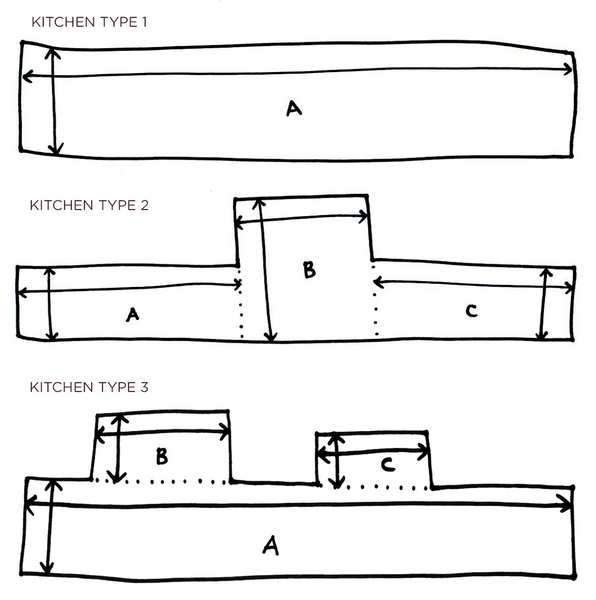Step 3 – Calculating the Coverage - How to calculate backsplash square footage?

Now that you have your measurements, it's time to calculate the coverage manually or with a backsplash calculator.  To measure how many tiles you'll need for your backsplash or any space, first figure out the entire space's area that you just measured. This will tell you the coverage (in inches) for each location that you want to tile.

To calculate your backsplash area, you need to know the width and height of your current backsplash. Once you have those measurements, simply multiply the width by the length to get the total square inches. Finally, divide that number by 144 to determine how many square feet of backsplash you need.

-For tiling on round areas of walls in your home, divide the total length you’ve measured by 2 to find the radius. Then multiply the radius by pi – or 3.14. Round it up to the nearest decimal again to get your total in square feet.

Here’s an example:

• Let's assume you want to tile a 20-foot-diameter circular space.
• Convert the measurement to inches: 20 feet = 240 inches
• To discover the radius, divide the diameter by two: 120 in / 2 = 60.
• To determine how much area you'll cover with your structure, multiply the radius by 3.14: 120 in * 3.14 = 376.8 sq ft.
• Round up to the nearest decimal: 377 square feet of coverage is the total area.

Pro Tip:

To figure out how much backsplash you need, you'll need to measure the square footage of your entire wall and subtract the square footage of any doors or windows. In this way,  you can account for the entire wall and the square footage of all doors and windows.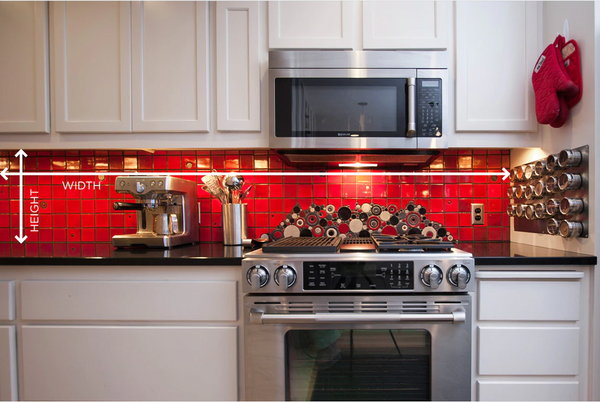Step 4 –  Calculate how much Backsplash tile does I need:

Aside from the total surface area, you may want to know how many tiles will be required to cover it. It's quite simple to convert the coverage area into a count of tiles.

To calculate the square footage of your backsplash, you will need to find the area of one tile. This can be done by multiplying the length and width of the tile in inches. Once you have this number, divide the area of your space by the area of one tile. This will tell you how many tiles you need to complete your backsplash.

Let's clear it with an example of how much backsplash tile do I need:

The item contains 10 sheets and each measures 11.8" x 11.8" | 30cm x 30cm. This means 1 pack covers 9.7 sq.ft / 0.90 m2. So, if you have 11.8" x 11.8" tiles and you want to wish to wrap a 48" wide by 24" high backsplash, you will need:

• To begin, calculate the total area of each tile: 11.8 in by 11.8 in = 140 in (rounded up)
• Second, determine how big your backsplash area is: 1,152 square inches.
• Finally, divide the space by one tile: 1,152 / 140 = 8.22 tiles required

We've compiled a list to make things simpler if you have square tiles! Simply choose your tile size from the list and use the calculation below to determine the minimal quantity of tiles you'll need. Make sure your total area is in square inches before proceeding!

• 4-inch tiles: Total Area ÷ 0.1089 = Number of 4″ tiles needed
• 6-inch tiles: Total Area ÷ 0.25 = Number of 6″ tiles needed
• 9-inch tiles: Total Area ÷ 0.5625 = Number of 9″ tiles needed
• 12-inch tiles: Total Area = Number of 12″ tiles needed
• 15-inch tiles: Total Area ÷ 1.5625 = Number of 15″ tiles needed
• 18-inch tiles: Total Area ÷ 2.25 = Number of 18″ tiles needed

If you're a non-mathematician, we have a backsplash calculator for each design so you can figure out how many tiles you'll need based on the total square footage from step 3.Overage is the amount of product you order to account for mistakes or cuts that may be necessary during the installation process.  Depending on the size and shape of your backsplash,  you will need between five and ten percent more than your total square footage to account for the overage.

We recommend adding five percent overage for small jobs under 100 square feet.  If your project is between 100 and 300 square feet,  add seven to eight percent overage.  For projects larger than 300 square feet,  add ten percent overage to your total square footage calculation.

Pro Tip:

If you were cautious and bought extra tiles, you may have some leftovers. Don't toss them away! It's a good idea to keep extra tiles on hand if you need to replace one in the future.3D Amber Clay Square Peel and Stick Tile for Kitchen Backsplash

## Final Measurement Tips for Specific Areas

While the basic method to measure a room for tile installation is fairly universal, there are a few things to watch out for when installing kitchen and bathroom tiles. We’ll take a look at these tips before we finish our guide!

• Measuring a Shower– When measuring a backsplash, it is important to take accurate measurements to calculate the square footage correctly. There are a few things to watch out for when measuring, such as niches and benches. If you are installing border tiles, be sure to measure the area's perimeter and divide by 12 to get the total linear feet needed. You can also use a backsplash calculator for calculations.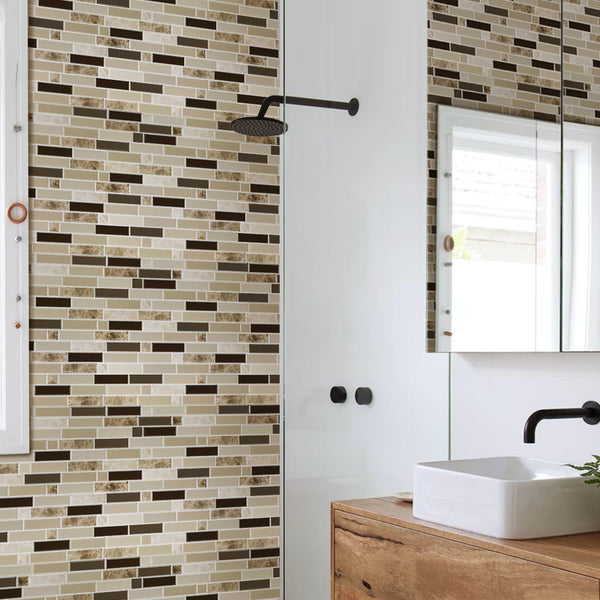Thicker Light Brown Mosaic Peel and Stick Tile for Shower Backsplash

• Measuring a Kitchen Backsplash:If you want to know how to measure a kitchen backsplash, you need to calculate the area of the entire wall you plan to cover. Subtract the area of any windows or other elements on the wall. It is easier to divide your space into smaller sections, such as left of a range, right of a range, and so on. A backsplash calculator can also help you calculate your backsplash.
• Measuring a Fireplace: When measuring a fireplace, you need to consider the width of the mantel and any overhang. You also need to measure the height from the floor to the top of the opening. To calculate square footage, multiply the width by the height. Calculations can also be done using a backsplash calculator.
• Measuring a Wall:To measure a wall, you need to calculate the area of the entire wall. Subtract the area of any windows or other elements on the wall. The backsplash calculator can also be used here.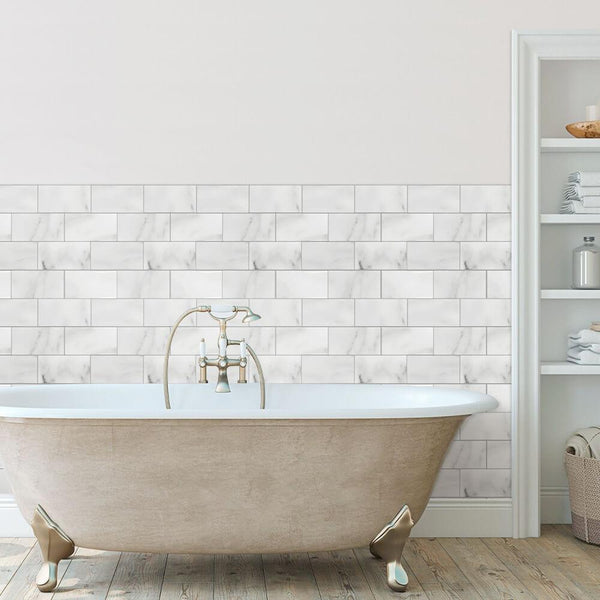Thicker Carrara Marble Subway Peel and Stick Tile for Shower Backsplash

## What Do You Need To Consider While Measure the Area of your Backsplash For Peel and Stick Tiles?

When measuring for a backsplash, there are a few things to keep in mind:  Some of these are:

Size of the stick tiles:

The size of the stick tiles will determine how many you need to cover a specific area. Normally, tiles come in 11.81” X 11.81”(30cmX30cm),  but the size may vary slightly from one manufacturer to another.

Layout:

The layout of your backsplash will also affect how many tiles you need.  If you plan on using a herringbone or other pattern, you will need more tiles than if you use a  straight layout.

The shape of your backsplash will also play a role in how many tiles you need.  If it is a simple square or rectangle, you can easily calculate the number of tiles needed. However,  if it is an oddly shaped space,  you may need some creative math to figure out how many tiles you will need or need of backsplash calculator.

Overage:

As with any project, it is always a good idea to order more tile than you think you will need.  This way,   you will have extras in case of breakage or make a mistake.  We recommend ordering between five and ten percent more than your total square footage to account for the overage.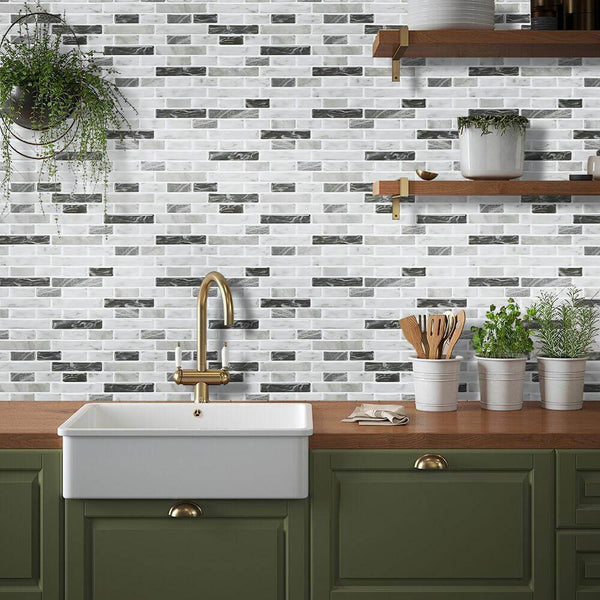Gray Tone Marble Mosaic Peel and Stick Tile for Kitchen Backsplash

Q:  Why Do You Divide By 144?

A:  This is because one square foot equals 144 square inches. So,   when you divide by 144,  you effectively calculate the number of square feet.

Q: Which way is the width on a wall?

A: The width is the distance between two opposite sides of a wall, measured from one corner.

Q:  What is a “straight layout”?

A: A straight layout is when you linearly install the tiles without any patterns or designs.  This is the simplest and most common type of backsplash installation.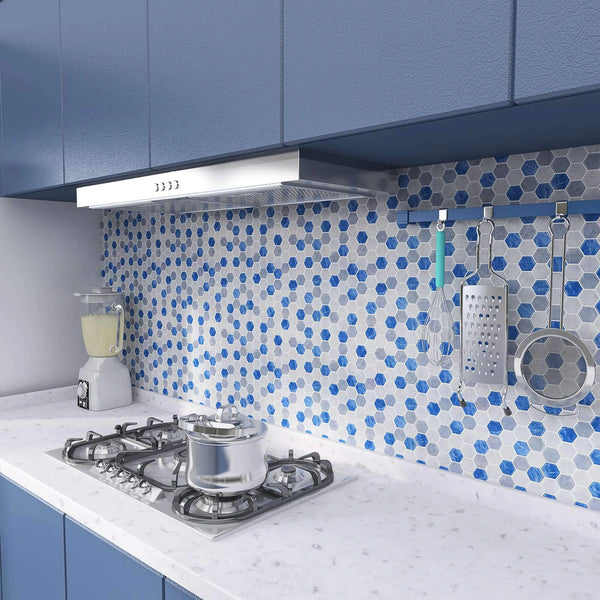Blue Marble Hexagon Peel and Stick Tile for Kitchen Backsplash

Q: How do I calculate the amount of backsplash material I need?

A: To calculate the amount of backsplash material you need, measure the length and width of the area you want to cover with backsplash material. Then, use a backsplash calculator to determine the total square footage of your area. Once you have the total square footage, use this number to calculate how much backsplash material you need.

Q:  Do I need to subtract anything from the wall area when measuring for a backsplash?

A: Yes,  you will need to subtract the area of any windows or other elements that are on the wall.  This will ensure that you have an accurate measurement of the space available for your backsplash

Q:  What if I am installing tile on a curved surface?

A: Curved surfaces can be a bit more difficult to measure.  We recommend using a flexible tape measure to get an accurate measurement.  You may also need to do

Q: What is the average size of a kitchen backsplash?

A: The average size of a kitchen backsplash is three to six inches up the wall, with four inches being mostly in height. However,  the size will vary depending on the dimensions of your kitchen.Green and Yellow Spanish Peel and Stick Tile for Bathroom Backsplash

## The Bottom Line: